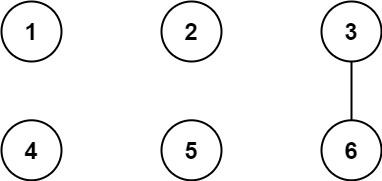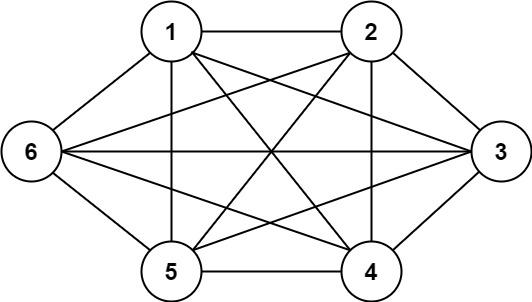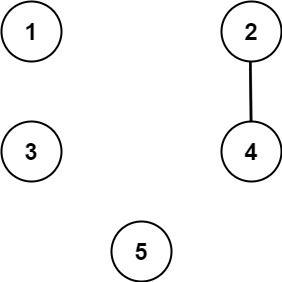# leetcode 1627. Graph Connectivity With Threshold （python）

「這是我參與11月更文挑戰的第28天，活動詳情檢視：2021最後一次更文挑戰

### 描述

We have n cities labeled from 1 to n. Two different cities with labels x and y are directly connected by a bidirectional road if and only if x and y share a common divisor strictly greater than some threshold. More formally, cities with labels x and y have a road between them if there exists an integer z such that all of the following are true:

• x % z == 0,
• y % z == 0, and
• z > threshold.

Given the two integers, n and threshold, and an array of queries, you must determine for each queries[i] = [ai, bi] if cities ai and bi are connected directly or indirectly. (i.e. there is some path between them).

Return an array answer, where answer.length == queries.length and answer[i] is true if for the ith query, there is a path between ai and bi, or answer[i] is false if there is no path.

Example 1:``````Input: n = 6, threshold = 2, queries = [[1,4],[2,5],[3,6]]
Output: [false,false,true]
Explanation: The divisors for each number:
1:   1
2:   1, 2
3:   1, 3
4:   1, 2, 4
5:   1, 5
6:   1, 2, 3, 6
Using the underlined divisors above the threshold, only cities 3 and 6 share a common divisor, so they are the
only ones directly connected. The result of each query:
[1,4]   1 is not connected to 4
[2,5]   2 is not connected to 5
[3,6]   3 is connected to 6 through path 3--6
``````

Example 2:``````Input: n = 6, threshold = 0, queries = [[4,5],[3,4],[3,2],[2,6],[1,3]]
Output: [true,true,true,true,true]
Explanation: The divisors for each number are the same as the previous example. However, since the threshold is 0,
all divisors can be used. Since all numbers share 1 as a divisor, all cities are connected.
``````

Example 3:``````Input: n = 5, threshold = 1, queries = [[4,5],[4,5],[3,2],[2,3],[3,4]]
Output: [false,false,false,false,false]
Explanation: Only cities 2 and 4 share a common divisor 2 which is strictly greater than the threshold 1, so they are the only ones directly connected.
Please notice that there can be multiple queries for the same pair of nodes [x, y], and that the query [x, y] is equivalent to the query [y, x].
``````

Note:

• 2 <= n <= 10^4
• 0 <= threshold <= n
• 1 <= queries.length <= 10^5
• queries[i].length == 2
• 1 <= ai, bi <= cities
• ai != bi

### 解析

• x % z == 0
• y % z == 0
• z > threshold

### 解答

``````class Solution(object):
def areConnected(self, n, threshold, queries):
"""
:type n: int
:type threshold: int
:type queries: List[List[int]]
:rtype: List[bool]
"""
father = {i:i for i in range(1, n+1)}
visited = {i:0 for i in range(1, n+1)}
for t in range(threshold+1, n+1):
if visited[t]:continue
for x in range(t, n+1, t):
visited[x] = 1
if self.getFather(x,father) != self.getFather(t,father): self.union(x, t,father)
result = []
for query in queries:
result.append(self.getFather(query,father)==self.getFather(query,father))
return result

def union(self,a,b,father):
a = father[a]
b = father[b]
if a<b: father[b] =  a
else: father[a] = b

def getFather(self,x,father):
if father[x] != x:
father[x] = self.getFather(father[x],father)
return father[x]
``````

### 執行結果

``````Runtime: 824 ms, faster than 100.00% of Python online submissions for Graph Connectivity With Threshold.
Memory Usage: 51.4 MB, less than 100.00% of Python online submissions for Graph Connectivity With Threshold.
``````